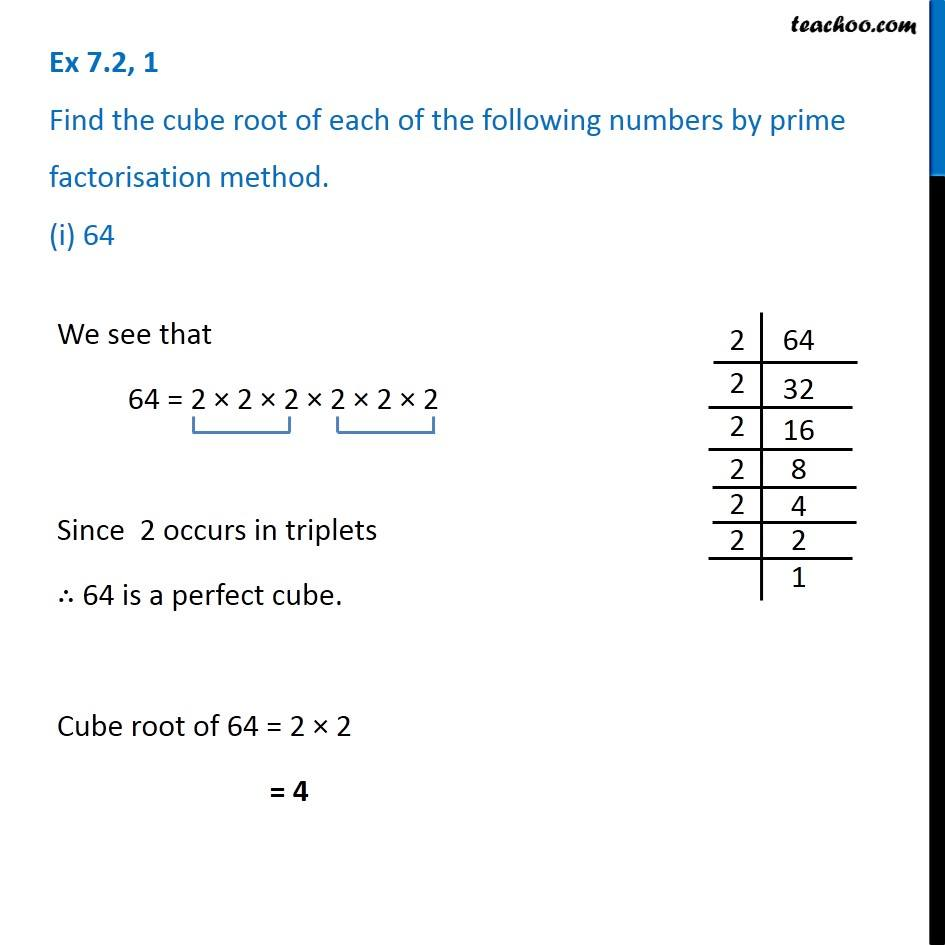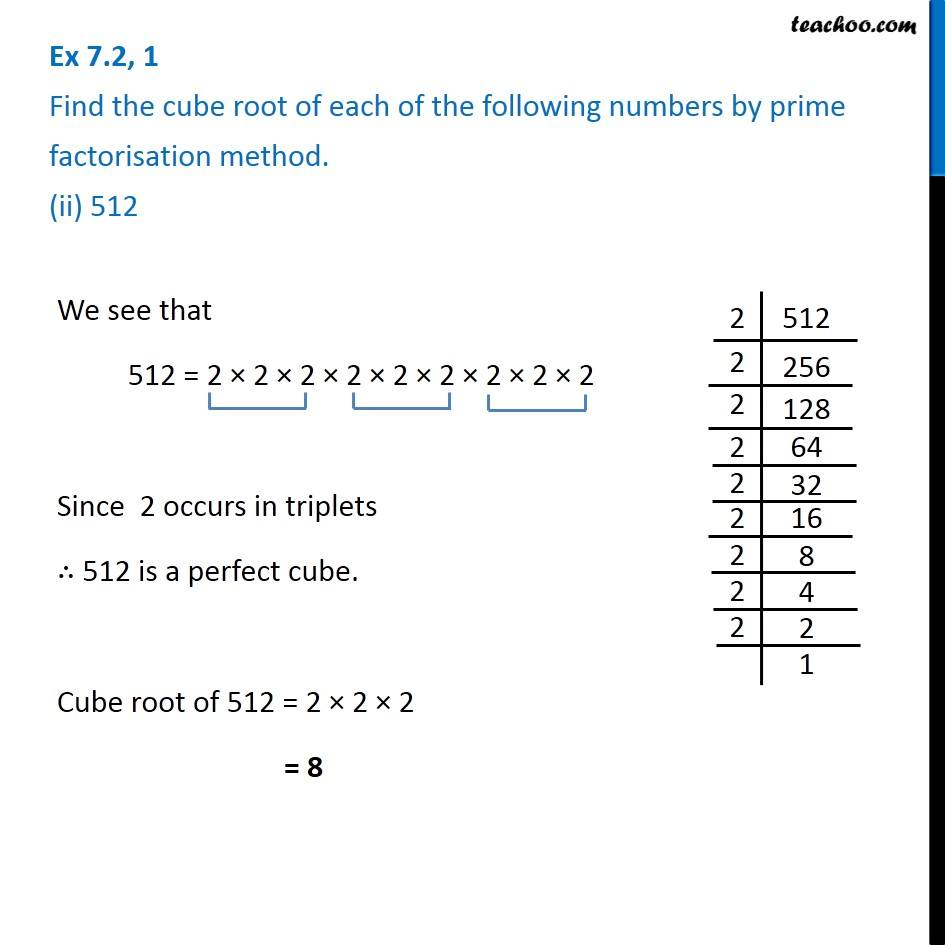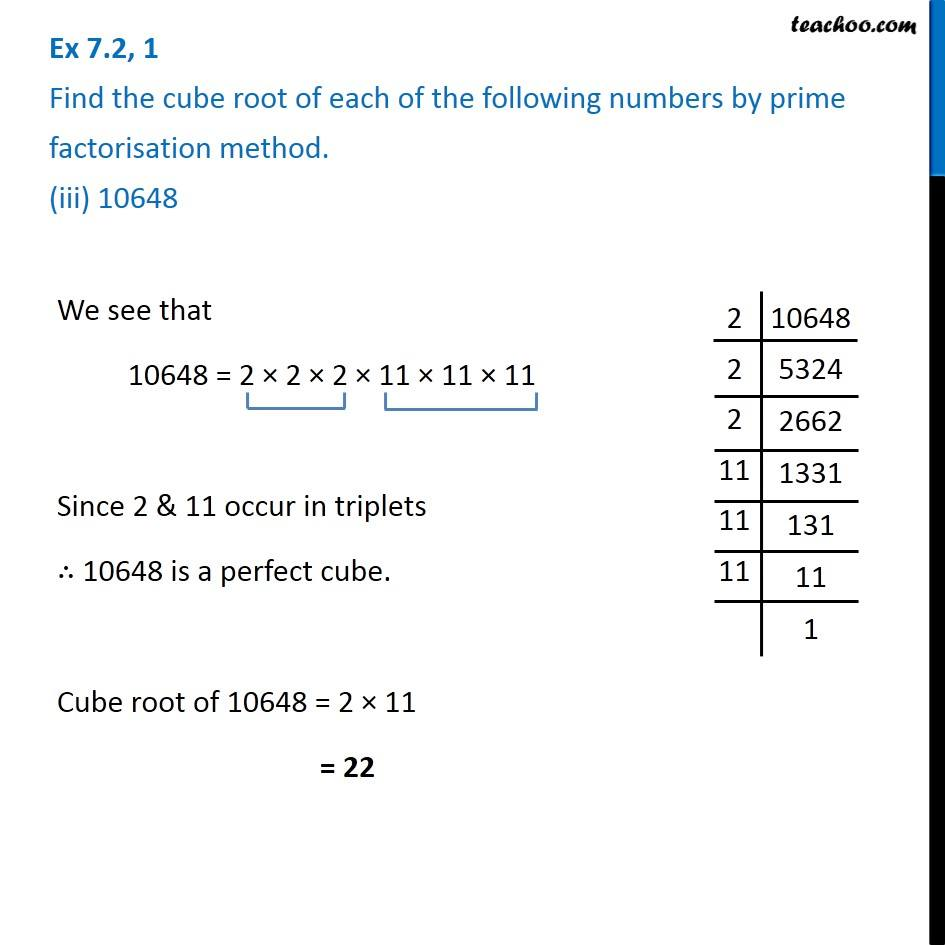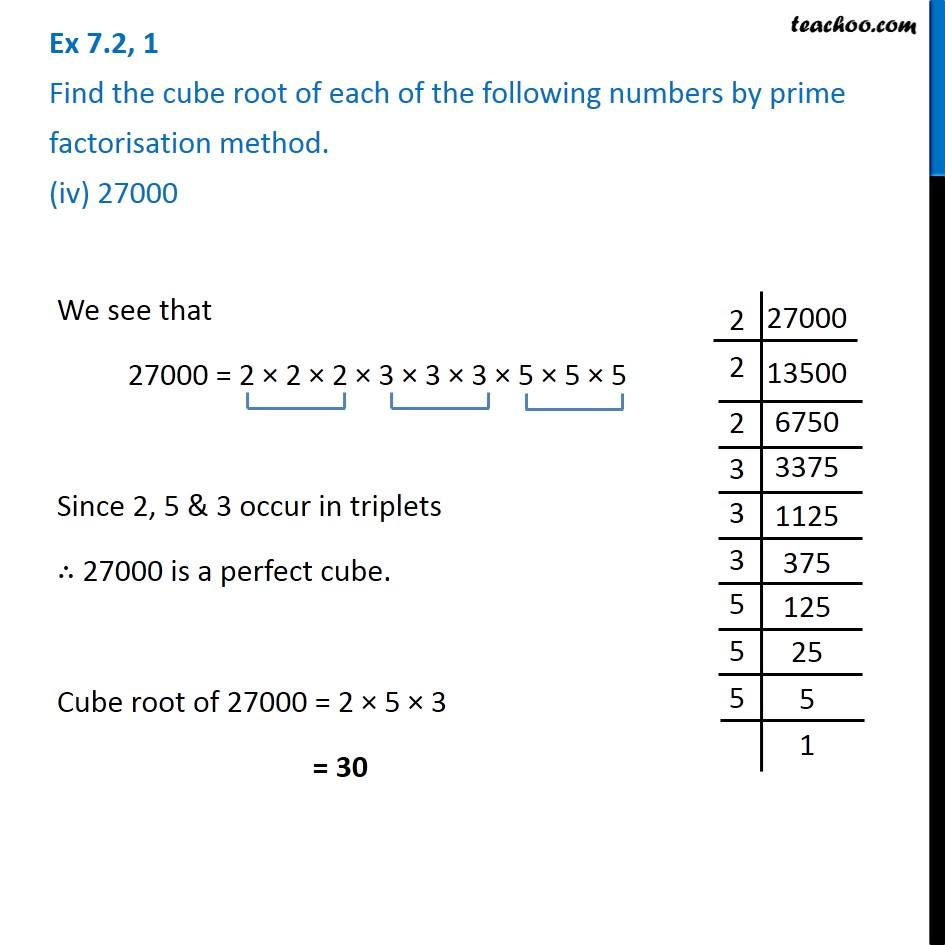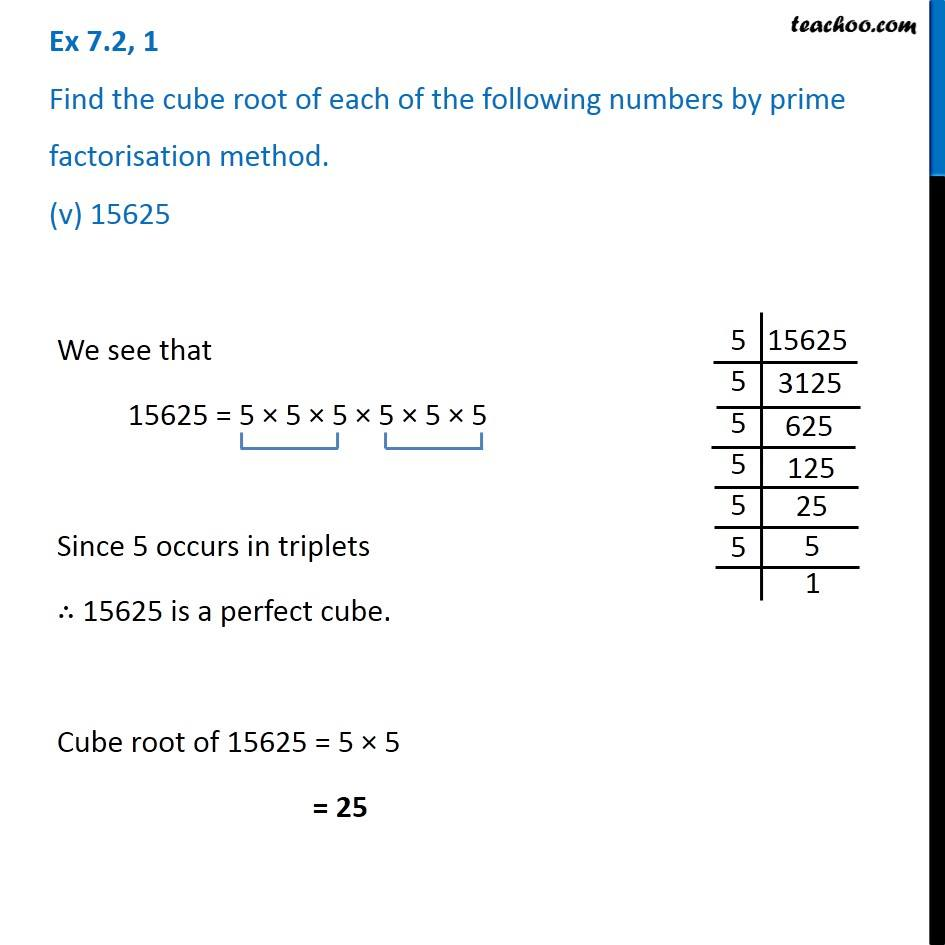1. Chapter 7 Class 8 Cubes and Cube Roots
2. Concept wise
3. Finding cube root by prime factorisation method

Transcript

Ex 7.2, 1 Find the cube root of each of the following numbers by prime factorisation method. (i) 64We see that 64 = 2 × 2 × 2 × 2 × 2 × 2 Since 2 occurs in triplets ∴ 64 is a perfect cube. Cube root of 64 = 2 × 2 = 4 Ex 7.2, 1 Find the cube root of each of the following numbers by prime factorisation method. (ii) 512We see that 512 = 2 × 2 × 2 × 2 × 2 × 2 × 2 × 2 × 2 Since 2 occurs in triplets ∴ 512 is a perfect cube. Cube root of 512 = 2 × 2 × 2 = 8 Ex 7.2, 1 Find the cube root of each of the following numbers by prime factorisation method. (iii) 10648We see that 10648 = 2 × 2 × 2 × 11 × 11 × 11 Since 2 & 11 occur in triplets ∴ 10648 is a perfect cube. Cube root of 10648 = 2 × 11 = 22 Ex 7.2, 1 Find the cube root of each of the following numbers by prime factorisation method. (iv) 27000We see that 27000 = 2 × 2 × 2 × 3 × 3 × 3 × 5 × 5 × 5 Since 2, 5 & 3 occur in triplets ∴ 27000 is a perfect cube. Cube root of 27000 = 2 × 5 × 3 = 30 Ex 7.2, 1 Find the cube root of each of the following numbers by prime factorisation method. (v) 15625We see that 15625 = 5 × 5 × 5 × 5 × 5 × 5 Since 5 occurs in triplets ∴ 15625 is a perfect cube. Cube root of 15625 = 5 × 5 = 25

Finding cube root by prime factorisation method